# GMAT Circles: An IntroductionAre you prepping for GMAT geometry questions? Feeling confused or in need of a refresher on circles? Read on to get a rundown of GMAT circles terminology, concepts, and practice questions.

## GMAT Circles: Basic Terminology

A circle is the set of all points equidistant from a fixed point.  That means a circle is this:and not this:Photo by cliparts.co

In other words, the circle is only the curved round edge, not the middle filled-in part.  A point on the edge is “on the circle”, but a point in the middle part is “in the circle” or “inside the circle.”  In the diagram below,point A is on the circle, but point B is in the circle.

By far, the most important point in the circle is the center of the circle, the point equidistance from all points on the circle.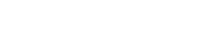## Chords

Any line segment that has both endpoints on the circle is a chord.By the way, the word “chord” in this geometric sense is actually related to “chord” in the musical sense: the link goes back to Mr. Pythagoras (c. 570 – c. 495 BCE), who was fascinated with the mathematics of musical harmony.

If the chord passes through the center, this chord is called a diameter.  The diameter is a chord.  A diameter is the longest possible chord.  A diameter is the only chord that includes the center of the circle.The diameter is an important length associated with a circle, because it tells you the maximum length across the circle in any direction.

An even more important length is the radius.  A radius is any line segment with one endpoint at the center and the other on the circle.As is probably clear visually, the radius is exactly half the diameter, because a diameter can be divided into two radii.  The radius is crucially important, because if you know the radius, it’s easy to calculate not only the diameter, but also the other two important quantities associated with a circle: the circumference and the diameter.## Circle Formulas

The circumference is the length of the circle itself.  This is a curve, so you would have to imagine cutting the circle and laying it flat against a ruler.  As it turns out, there is a magical constant that relates the diameter (d) & radius (r) to the circumference.  Of course, that magical constant is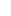.  From the very definition ofitself, here are two equations for the circumference, c.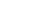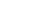If you remember the second, more common form, you don’t need to know the first.  The numberis slightly larger than 3 — this means that three pieces of string, each as long as the diameter, together would not be quite long enough to make it all the way around the circle.  The numbercan be approximated by 3.14 or by the fraction 22/7.  Technically, it is an irrational number that goes on forever in a non-repeating pattern.

These two formulas follow from the definition of, so basically every culture on earth figured out these.  By contrast, the area of circle was discovered by one brilliant mathematician, and everyone on earth has this one man to thank for his formula for the area of a circle.  That man was Archimedes (c. 287 – c. 212 BCE).  Here is Archimedes’ amazing formula: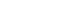• This is a GMAT circle formula you need to know cold on test day.## Circles and Angles

Suppose you stand at the center of a circle and turn around that you face each and every point on the circle.  You would turn all the way around, which is an angle of 360º.  In this sense, a whole circle has an angle of 360º.  If you divided a circle equally, you could calculate the angle of each “slice”.  Here are a few division results that could help you to know on test day (I’m just giving the ones that come out as nice round numbers, not the ones that result in ugly decimals):

360/2 = 180

360/3 = 120

360/4 = 90

360/5 = 72

360/6 = 60

360/8 = 45

360/9 = 40

360/10 = 36

360/12 = 30

### Arcs and Arclength

Suppose we look at a “slice” of a circle, like a slice of pizza.The curved line from A to B, a part of the circle itself, is called an arc.  This corresponds to the crust of the pizza.

We can talk about the size of an arc in one of two ways: (a) its angle, sometimes called “arc angle” or “arc measure“, and (b), its length, called arclength.  The angle of the arc, its arc measure, is just the same as the angle at the center of the circle.  Here ∠AOB = 60°, so the measure of arc AB is 60°.

We find the arclength by setting up a proportion of part-to-whole.  The angle is part of the whole angle of a circle, 360°.  The arclength is part of the length all the way around, i.e. the circumference.  Therefore: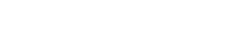Here, let’s say the radius is r = 12.  Then, the circumference is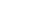.  Since the angle is 60°, the ratio on the left side, angle/360, becomes 1/6.  Call the arclength x.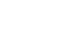Cross-multiply: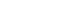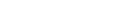In other words, since the angle 60° is one sixth of the full angle of a circle, the arclength is one sixth of the circumference.## GMAT Circles Practice Questions1. In the shaded region above, ∠KOL = 120°, and the area of the entire circle is.  The perimeter of the shaded region is
1.2.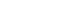3.4.5.1) The area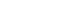, so r = 12.  This means KO = 12 and OL = 12, so those two sides together are 24.  The remaining side is arc KL.  The whole circumference is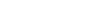.  The angle of 120° is 1/3 of a circle, so the arclength is 1/3 of the circumference.  This means,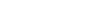, and therefore the entire perimeter is.  Answer = C.

1. Given that a “12-inch pizza” means circular pizza with a diameter of 12 inches, changing from an 8-inch pizza to a 12-inch pizza gives you approximately what percent increase in the total amount of pizza?
1. 33
2. 50
3. 67
4. 80
5. 125

The 8-inch pizza has a radius of r = 4, so the area is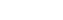. That area is how much pizza you get. The 12-inch pizza has a radius of r = 6 and an area of. When you change from 16 to 36, what is the percentage change? Well, that’s more than double, so it must be a percent greater than 100%. The only answer choice greater than 100% is answer E.

1. What is the diameter of circle Q?
• Statement #1 — the circumference of Q is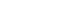.
• Statement #2 — the area of Q is.

Statement #1: if you know the circumference, then you can use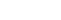to solve for the radius, or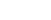to solve for the diameter. Either way, you can find the diameter, so this statement by itself is sufficient.

Statement #2: if you know the area, you can find the radius, and then double that to get the diameter. This statement by itself is also sufficient.

Both statements alone are sufficient. Answer = D.

•